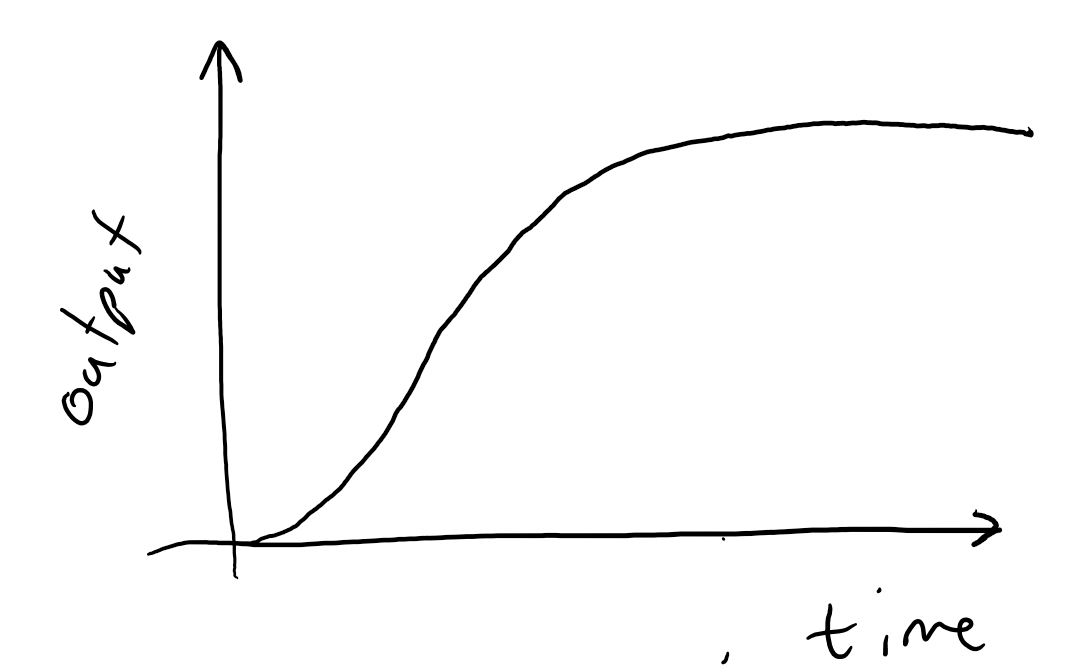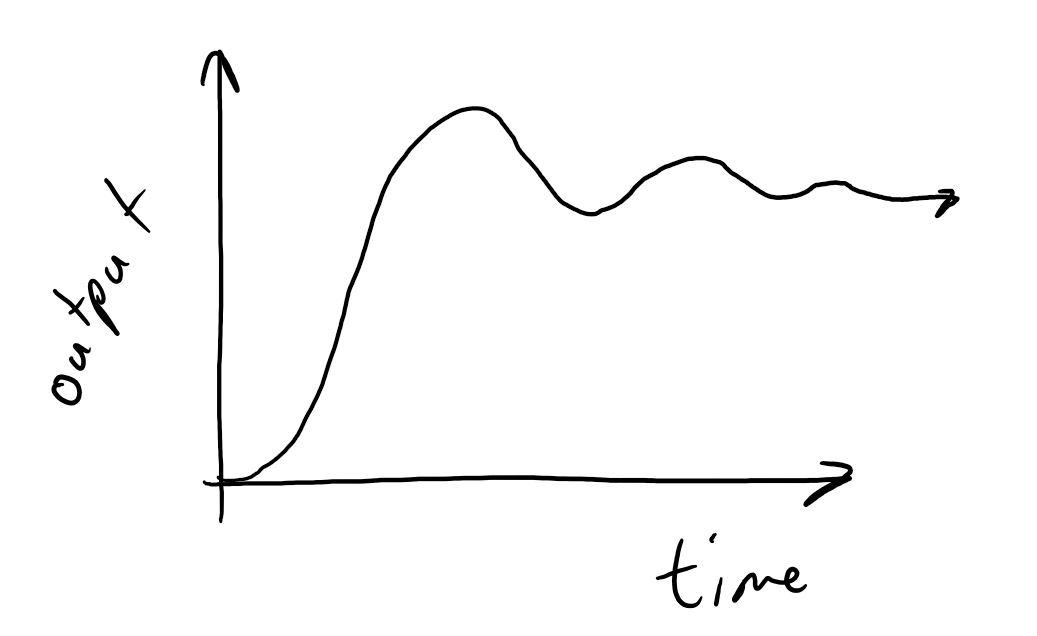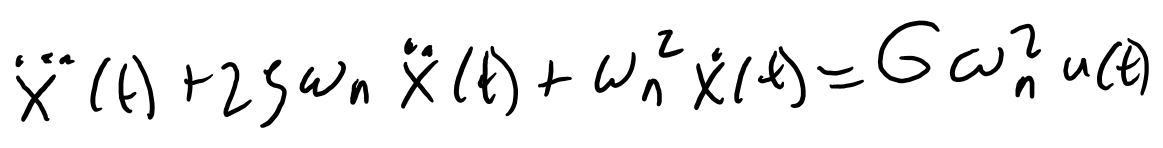Robotics 1
Checkpoint 3: Motion ControlExample Question 2:  You connect the motor in Question 1 to a motor driver chip like we used in class, and you use an external power supply that is 12V.  Then, you set the PWM period to 100, you set the compare value to 100, and observe that the motor is turning 94rpm.  Write an equation to calculate the compare value that will get the motor to turn a desired speed.
Example Question 3:  Now, suppose that you apply a constant 12V supply to your motor from Question 1.  You exert a force on the motor shaft until it stops.  You observe that you need to exert 0.5Nm of torque to make the motor stop.  Draw a torque-speed curve for this motor at 12V.
Example Question 4: Using your torque-speed curve from Question 4, can the motor lift a 0.5kg mass attached to a pulley with a radius of 5cm when you are providing 12V to the motor?  If so, how fast can the motor lift the mass?
Example Question 5:  What are the differences between open-loop control and feedback control?  Why might we use each type of control in different circumstances?
Example Question 6: Shown here on the left are three kinds of control we learned about in this unit.  On the right is a list of possible properties of a control algorithm.  Draw lines to indicate which properties are true of which types of control.  Each item on the left can be matched to multiple items on the right, and vice-versa.
Example Question 7: If I increase the value of Kp, what will be the effects on speed, stability, and accuracy of the control?
Example Question 1: You have a motor for which you don't have a datasheet.  You don't connect the motor shaft to anything, and you connect the motor leads to a 6V power supply.  You measure the speed of the motor to be 47rpm.  How fast would you expect the motor to turn if you increase the voltage to 8V?
Congratulations!  You've made it all the way through our third unit: Motion Control.  Before we move on, you will need to take a test to make sure that all this information has sunk in.  Here are some example problems like the ones you will see on the test in class.  On our review day (the class day before the test), I'll go through these problems live on the board.  So, it's a good idea to try to solve the problems on your own before coming to class.
Example Question 8: I am using UART to send the number 82, and I have set a baud rate of 38400.  Also, I have set one start bit and one stop bit.  Draw the voltage vs time plot that we would expect to see during this transmission.  How long does it take to send one bit?  How long does it take to complete the transmission?
Example Question 9: Shown here is a step response I captured while moving my rack and pinion.  Find values for the rise time, peak time, settling time, overshoot (percent), and steady-state error.Example Question 10: What does it mean for a response to be 'critically-damped'?Graduates-Only Example Question 1: Listed on the left are three possible system 'orders'.  Listed on the right are equations and step response plots.  Draw lines to match the items on the left with the items on the right.  Each item might have zero, one, or more than one match, and each item on the right might have zero, one, or more than one match.
Graduates-Only Example Question 3:  Use your answers from Example Question 10 to find the damping ratio and natural frequency for the system.  Write the final differential equation that models the system.
Graduates-Only Example Question 4:  Suppose that the response in Example Question 10 was obtained after setting Kp = 1 in a closed-loop system.  Find the value of Kp that would give a critically-damped system.
Open-loop control

On-off control

Proportional control
- Requires a sensor
- Is able to reject disturbances
- Is always stable
- Is a type of feedback control
- Is able to hold a position
- Varies the speed of the motor based on distance from the target position
- Has a 'gain' that you, the user, can select to change the speed, accuracy, and stability of the response
First-order

Second-order

Third-orderFirst-order

Second-order

Third-order
Graduates-Only Example Question 2: Listed on the left are three possible system 'orders'.  Listed on the right are equations and step response plots.  Draw lines to match the items on the left with the items on the right.  Each item might have zero, one, or more than one match, and each item on the right might have zero, one, or more than one match.